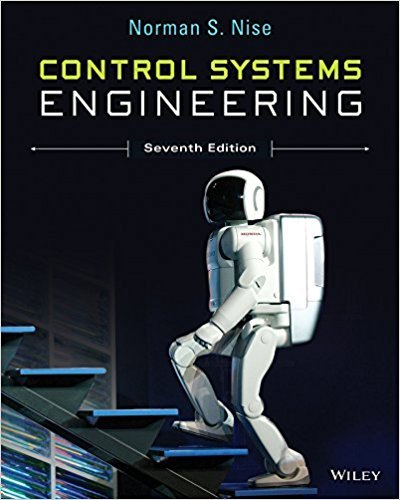×
Get Full Access to Control Systems Engineering - 7 Edition - Chapter 1 - Problem 2
Get Full Access to Control Systems Engineering - 7 Edition - Chapter 1 - Problem 2

×

# A temperature control system operates by sensing theISBN: 9781118170519 162

## Solution for problem 2 Chapter 1

Control Systems Engineering | 7th Edition

• Textbook Solutions
• 2901 Step-by-step solutions solved by professors and subject experts
• Get 24/7 help from StudySoup virtual teaching assistantsControl Systems Engineering | 7th Edition

4 5 1 329 Reviews
23
0
Problem 2

A temperature control system operates by sensing the difference between the thermostat setting and the actual temperature and then opening a fuel valve an amount proportional to this difference. Draw a functional closedloop block diagram similar to Figure 1.8(d) identifying the input and output transducers, the controller, and the plant. Further, identify the input and output signals of all subsystems previously described. [Section 1.4: Introduction to a Case Study]

Step-by-Step Solution:
Step 1 of 3

CS 70 Discrete Mathematics and Probability Theory Spring 2016 Rao and Walrand Note 2 1 Proofs In science, evidence is accumulated through experiments to assert the validity of a statement. Mathematics, in contrast, aims for a more absolute level of certainty. A mathematical proof provides a means for guar- anteeing that a statement is true. Proofs are very powerful and are in some ways like computer programs. Indeed, there is a deep historic link between these two concepts that we will touch upon in this course — the invention of computers is intimately tied to the exploration of the idea of a mathematical proof about a century ago. So what types of “computer science-related” statements might we want to prov

Step 2 of 3

Step 3 of 3

##### ISBN: 9781118170519

This textbook survival guide was created for the textbook: Control Systems Engineering, edition: 7. This full solution covers the following key subjects: difference, temperature, output, Input, Introduction. This expansive textbook survival guide covers 13 chapters, and 734 solutions. The answer to “A temperature control system operates by sensing the difference between the thermostat setting and the actual temperature and then opening a fuel valve an amount proportional to this difference. Draw a functional closedloop block diagram similar to Figure 1.8(d) identifying the input and output transducers, the controller, and the plant. Further, identify the input and output signals of all subsystems previously described. [Section 1.4: Introduction to a Case Study]” is broken down into a number of easy to follow steps, and 69 words. The full step-by-step solution to problem: 2 from chapter: 1 was answered by , our top Engineering and Tech solution expert on 11/23/17, 05:05AM. Since the solution to 2 from 1 chapter was answered, more than 952 students have viewed the full step-by-step answer. Control Systems Engineering was written by and is associated to the ISBN: 9781118170519.

Unlock Textbook Solution